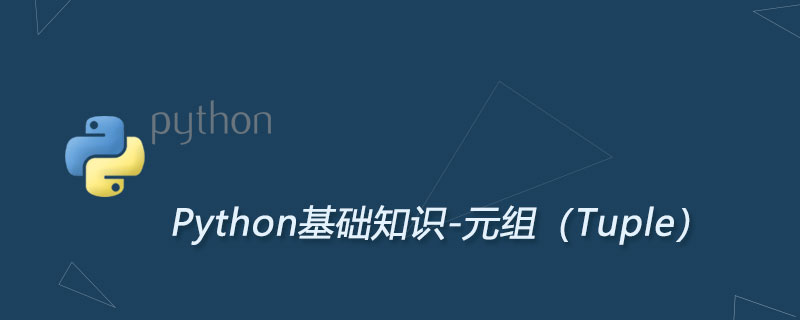python元组数据类型讲解colors  =  ('red',  'green',  'blue')
print("The first color is: " + colors)

print("\nThe available colors are:")
for color in colors:
print("- " + color)

colors = ('red', 'green', 'blue')
colors.append('purple')

animal = 'dog'
print("I have a " + animal + ".")

animals = ['dog', 'cat', 'bear']
for animal in animals:
print("I have a " + animal + ".")

Python 通常会忽略掉我们放进字符串的字符，但是它会对少许字符格外注意。例如 "\t" 和 "\n"。Python 同样也会注意到 "%s" 和 "%d"。它们被称为占位符。当 Python 看到了 "%s" 占位符，它就会找到 % 后的第一个参数填充进占位符。如下所示：

animal = 'dog'
print("I have a %s." % animal)

animals = ['dog', 'cat', 'bear']
print("I have a %s, a %s, and a %s." % (animals, animals, animals))

number = 23
print("My favorite number is " + number)

number = 23
print("My favorite number is " + str(number) + ".")

number = 23
print("My favorite number is %d." % number)

numbers = [7, 23, 42]
print("My favorite numbers are %d, %d, and %d." % (numbers, numbers, numbers))

Python开发开发项目